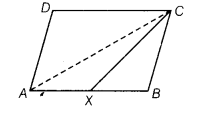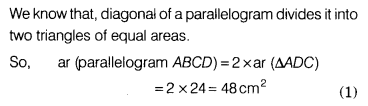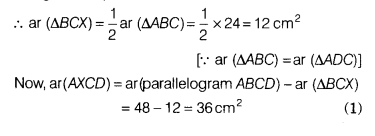# ABCD is a parallelogram and X is the mid-point of AB. If ar (∆ADC) = 24cm 2, then find ar (AXCD)

ABCD is a parallelogram and X is the mid-point of AB. If ar (∆ADC) = 24cm 2, then find ar (AXCD).

Given, ABCD is a parallelogram and X is the mid-point of AB. Draw the diagonal AC.We know that, diagonal of a parallelogram divides it into two triangles of equal areas.Since, CX is the median of AABC.

We know that a median of a triangle divides it into two triangles of equal areas.